# Gaussian Mixtures to Model Real Estate Prices in the UK

Synthetic datasets are boring. In most cases, we just generate them by drawing samples from a multivariate distribution with components from families of distributions that we have set previously, adding some noise at the end to make them more realistic. And of course, modelling a synthetic dataset is rather easy, as we have access to the underlying distribution of the data.

Real datasets are much more exciting. We are given some data and the task of modelling their underlying distribution. There are no right or wrong answers, and every approach is fair game. There are obviously some better and worse solutions which is precisely what we try to explain in this post.

Consider this dataset from UK’s HM Land Registry, hosted in Kaggle. It contains more than 22M real estate transactions for the period between 1995 and 2016, with individual information on the price of sale, date, location and type of property, among others. From the original dataset, we select the period between 2012-2014 and consider only the columns that describe the date of sale and price.

# 1. Objective and Approach

Our aim is to model the probability distribution of prices paid for real estate in the selected period. We examine four different approaches to tackle this problem:

• Gamma fit
• Log-normal fit
• Gaussian Mixture for the log-price data
• Gaussian Mixture

In this post, we review mixture models  to show how these can be useful when dealing with complex probability distributions. In particular, we apply a Gaussian mixture model to model the probability distribution of prices. The code to run these simulations can be found on github.

What worked? Gaussian Mixtures provided a great balance between mathematical complexity, difficulty in the implementation and results for the fit.
What didn’t work? I initially tried fitting a mixture of Gammas because I thought it might give better results. This became a mathematical nightmare that I quickly dropped, sticking with the nice closed-form results for Gaussian mixtures.
What was time-consuming? I spent a significant part of the time understanding and interpreting the components in each GM fit, as well as finding a nice way to demonstrate my insights. As a result, I decided on the log-log, log-x and linear plots to show each part of the distribution in detail.
Why should we care about this? Modelling the underlying distribution is crucial to understanding our data. Although in many situations we might get away with a known distribution, this is not always the case, as we have clearly shown. Having mixture models in our toolbox allows us to work with more complex datasets.

Let’s examine the evolution of the mean daily price paid per property in the selected period.

It is very clear that there is an increasing trend in this time series. The series starts with mean values around 230k and ends with mean values in the order of 280k, implying an increase of around 20%. Before modelling the probability distribution for the whole series, we adjust the prices for this increase.

We model the trend using an exponential function of the form

where a, b are the parameters we need to fit, t is the time (in days) since the beginning of 2012 (our selected period) and y is the target price (in £). The result of the fit is given below.

An exponential fit provides a model with an intuitive interpretation in this context, where we have a geometric growth (similar to inflation) of prices. Note that a linear fit would give a very similar result but would lack such interpretability.

To adjust the prices, we multiply the price of the sale by exp(-bt), where b is the parameter obtained from the fit and t is the number of days between the beginning of 2012 and the actual day of the sale. As a result, we obtain an adjusted time series that looks as follows:

This result is far from perfect because we still observe periods where the adjusted price goes far away from the mean (which corresponds to parameter a from our exponential fit). However, it is a significant improvement with respect to our original data because we have eliminated its trend.

# 3. Modelling the Price using a known Distribution

We’ll assume the exponential adjustment is sufficiently good for our analysis. Now let’s examine the distribution of adjusted prices.

This histogram has been truncated to prices below £1.2M and grouped in bins of £1k. The price distribution is relatively smooth and has a heavy tail with a maximum above £80M (not shown in the histogram).

As a first approach, we model the probability distribution using a Gamma. Note the pdf for this distribution is given by

where α, β and x₀ are the parameters to be estimated. It turns out that for our data a Gamma distribution is not a good model. The Maximum Likelihood Estimation (MLE) with the entire distribution does not give anything meaningful so we don’t include that result here. The fits with percentiles 95 and 99 (p95 and p99) of the data are given below.

The p95 fit captures well the mode of the empirical distribution but it does not explain a significant proportion of the data. As we try to include a larger part of the tail, the model breaks. We see that for p99, the match between the empirical and Gamma fitted distributions is much worse than for p95.

In conclusion, the Gamma distribution is poor at capturing the heavy tail of our data.

The histograms for the price (with log-x axis) are given below. For the plot on the left, we have also used a log-y axis to clearly show the heavy tail of the distribution, with prices above 1M for tens of thousands of transactions.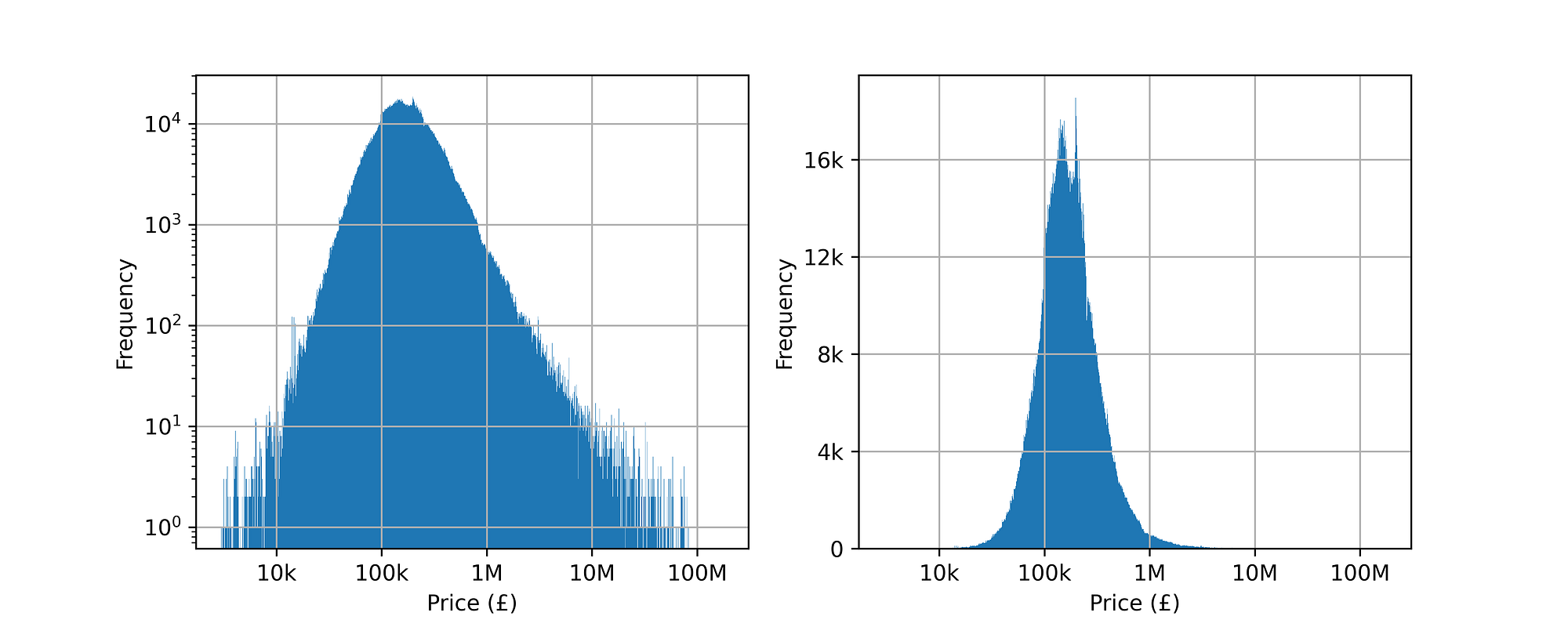(left) Log-log histogram for price data; (right) Log-x histogram for price data.

The plot on the right resembles a Gaussian distribution which motivates using a log-normal distribution to model the prices. To this end, we transform our original price distribution X as

where

is distributed as a Gaussian with mean μ and standard deviation σ. We compute the MLE fit with the log transformation of the data and obtain the following results.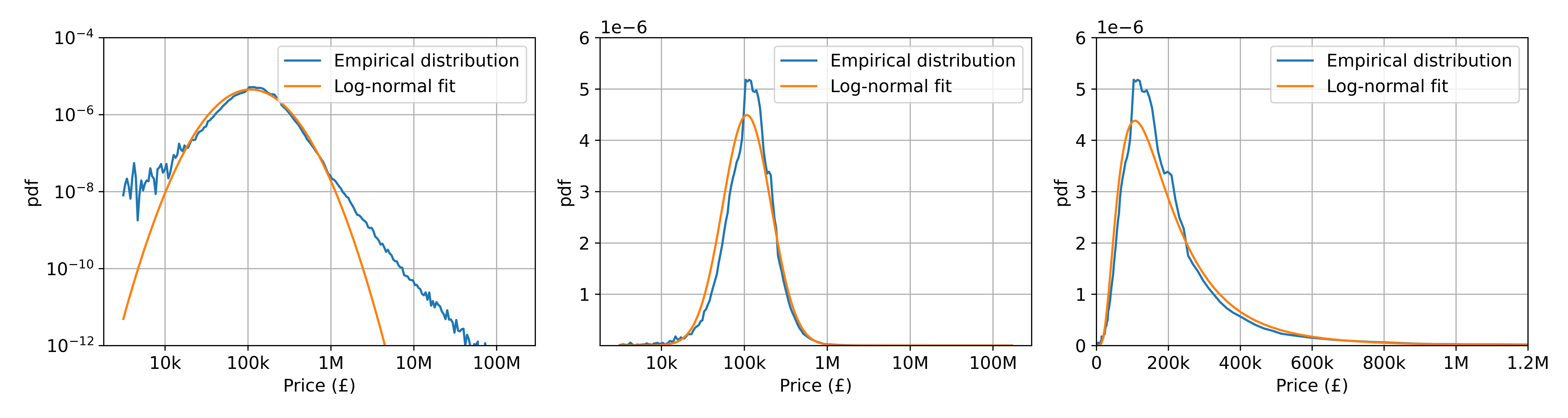(left) Log-log pdf; (centre) Log-price pdf; (right) pdf truncated at 1.2M.
• The plot on the left shows the log-log pdf for both the empirical distribution (i.e, the data) and the log-normal fit. We observe that for selling prices larger than £1M, the fit systematically underestimates the observed probability.
• The centre plot shows the log-x pdf. The log-normal fit captures correctly the mode at around £100k. However, we see that it underestimates the probability allocated at the mode. We also observe this in the linear-x plot on the right.

We are interested in finding a model that can approximate better the pdf both at the mode and the tail of the distribution. One way to do this consists of using mixture models which we review next.

# 4. Modelling the Price using Gaussian Mixtures

As we saw, a log-normal fit for our property prices data presents certain limitations, since the two free parameters that we fit in the MLE cannot capture all the complexity present in the data. Mixture models provide a solution to this problem. We assume that our data X is distributed as the linear combination:

where the weights ωₖ satisfy

and the basis functions gₖ are probability density functions (pdf) from known families of distributions. In this case, we choose gₖ to be Gaussian, therefore:

Instead of estimating (μ, σ) as we did before, now we estimate (ωₖ, μₖ, σₖ) for all components in the mixture. In the numerical experiments below, we have used the Expectation-Maximisation (EM) algorithm to obtain the parameters for the mixture which is very simple and easy to implement.

We set the number of components in the mixture K = 6 and find the MLE solution for a Gaussian Mixture (GM) for Y = log(X), where X is the adjusted real estate prices. This value has been chosen heuristically to strike a good balance between the number of parameters (model complexity) and the capacity of the model to explain the data. We briefly address how to quantitatively select the number of components in the mixture in “Open Questions”.

We set K = 6 and find the MLE solution for a Gaussian Mixture (GM) for X, the adjusted real estate prices.

# 5. Results

So far we have discussed four approaches to model the probability distribution of real estate prices, namely:

• Gamma fit
• Log-normal
• Gaussian Mixture for the log-price data
• Gaussian Mixture

We discard the Gamma fit from further analysis due to its severe limitations and compare the remaining three. In the graph below, we have plots with four lines, one corresponding to the empirically observed distribution (data pdf) and one for each of our fitted models.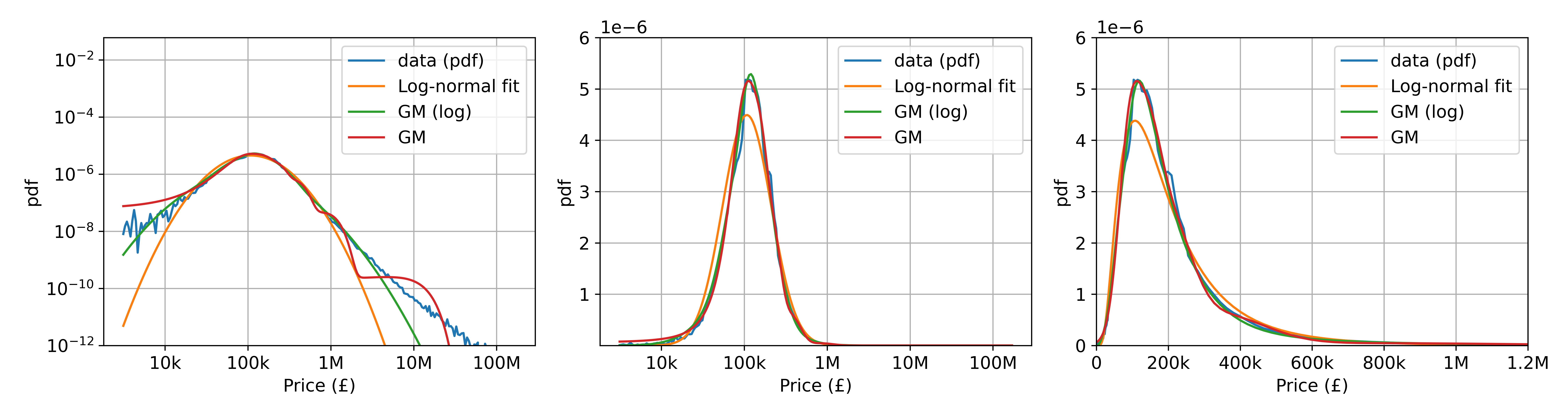(left) Log-Log pdfs; (centre) Log-x pdfs; (right) Pdfs truncated at 1.2M.

As we already analysed the log-normal fit, let’s discuss the GM for the log-price (green curves). This fit is clearly better. In the (left) log-log plot we observe that the GM-log fit is closer to the empirical pdf than the log-normal fit. Additionally, in the centre and right plots, we note that the mode is not only captured but also assigned the correct probability. The only limitation of this mixture is that it systematically underestimates probabilities for prices above £5M.

The GM fit for the price gives slightly different results. It is also preferable to the log-normal fit since it follows the tail of the distribution for large prices, and it assigns the mode the correct probability, as we see in the centre and right plots. However, we also observe that at the head and the tail of the distribution, there is still a discrepancy to the empirical pdf.

In both GM fits we chose K=6 components. We now have a look at how these components look and contribute to the final GM distribution.

In the graphs below, we have plotted a line for each component gₖ in the GM log fit, weighted by its corresponding ωₖ, each denoted by Cₖ for k=1…K (not sorted in any particular order).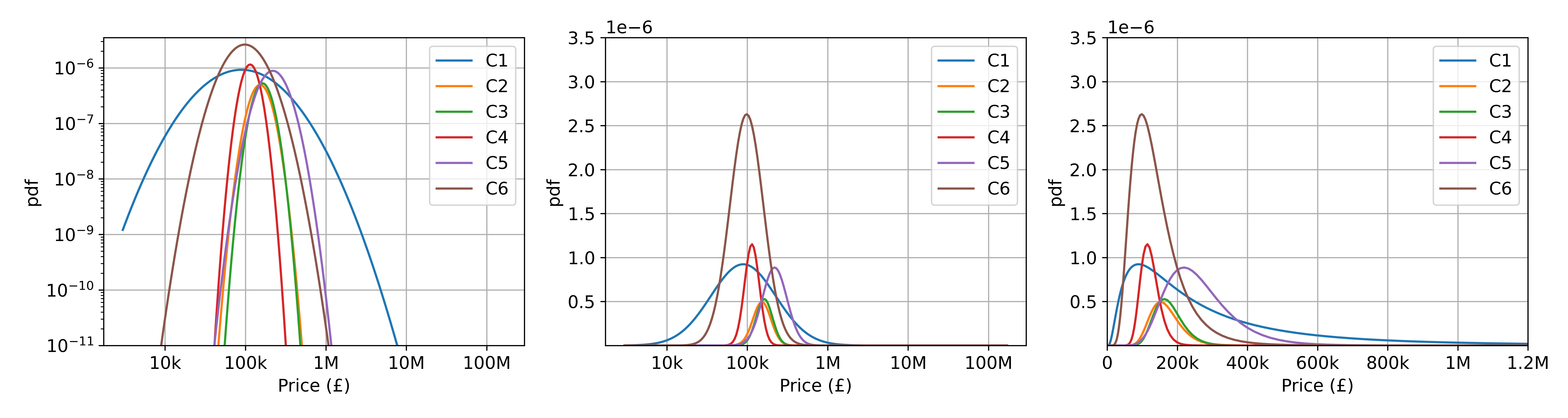[GM log-price components] (left) Log-log pdfs; (centre) Log-x pdfs; (right) Pdfs truncated at 1.2M.

These plots are quite revealing. The (left) log-log plot shows that the components present a very sharp decrease at the tail of the distribution which is why this mixture struggles to capture probabilities for large prices. The plot on the right makes explicit that each component is a log-normal distribution in the linear space (as expected).

Similarly, we analyse the components for the GM fit for the price. In the graph below, we have plotted the pdf for the weighted components ωₖgₖ, each denoted by Cₖ.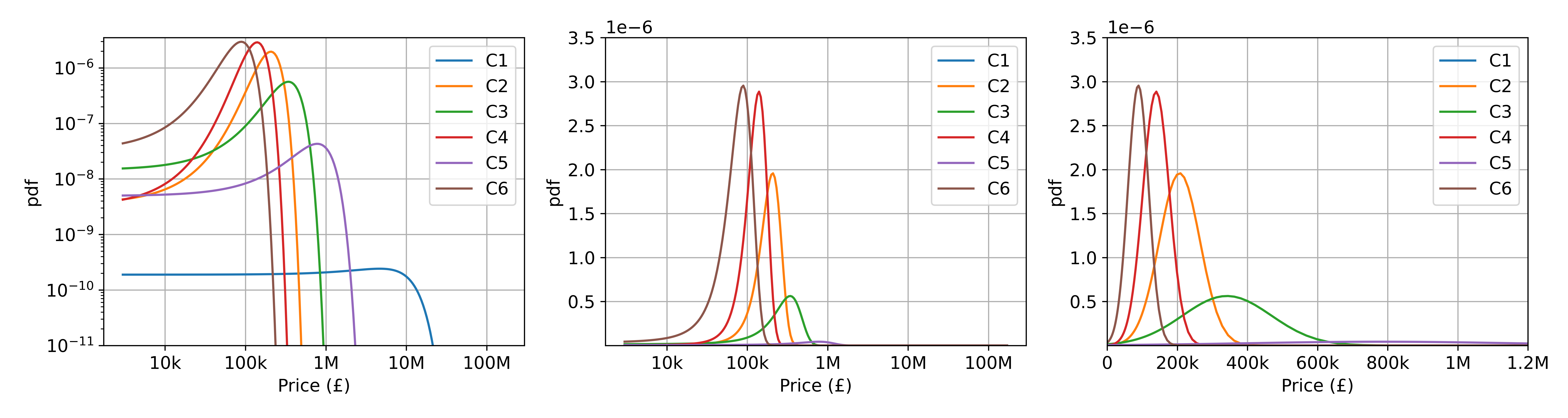[GM price components] (left) Log-log pdfs; (centre) Log-x pdfs; (right) Pdfs truncated at 1.2M.

The plot on the right shows that the pdf is decomposed using Gaussians in the linear space. In the plot on the right, we see why the pdf has wiggles, as we have a component (C1) with a very large mean (more than £5M) with a very small weight which contributes to allocating probability at the tail of the distribution. This is something we did not have either in the log-normal or the GM-log fits, as these fits systematically underestimated the probability at the tail of the distribution.

Additionally, the GM fit assigns a non-zero probability to relatively small, non-negative values, which are unrealistic (all price values in the dataset are strictly positive, as expected). However, for consistency, we have excluded prices lower than zero from the linear-x plot on the right.

There are different directions for improving this analysis. A fair question would be: how do we choose between models? As of now, We do not have a clear answer on whether the GM-log or GM (or even the log-normal) fit is preferred. We discussed the results qualitatively but did not provide a metric to make a quantitative decision. Additionally, we could ask how best to choose the number of components. Both these questions can be answered via the Bayesian Information Criterion (BIC) which allows choosing between models with a different number of parameters via computation of the likelihood.

# Summary

• Mixture Models provide a flexible framework to model complex distributions coming from real data.
• We used Gaussian Mixtures (GM) to model the distribution of real estate prices in the UK for the period 2012–2014. We compared GMs against more simple distributions such as Gamma or Log-normal which provided worse results for the fit.
• Gaussian Mixtures are simple and easy to implement in combination with the EM algorithm. There are analytical expressions for estimating all parameters which is not the case for other mixtures.

# References

 McLachlan, Geoffrey J., Sharon X. Lee, and Suren I. Rathnayake. “Finite mixture models.” Annual review of statistics and its application 6 (2019): 355–378.

Would you like to understand how AI can generate value for your business? Visit DataSparQ to find out more about the products we could build for you and the services we offer or get in touch with a member of the team to start your AI journey today.

## Datasparq Technology

Welcome to Datasparq’s technology blog.

## Datasparq Technology

Welcome to Datasparq’s technology blog. At Datasparq, we design, deliver and run bespoke data science products to help organisations capture value from data.

Written by

## Kiko Rul·lan

Data Scientist at DataSparQ## Datasparq Technology

Welcome to Datasparq’s technology blog. At Datasparq, we design, deliver and run bespoke data science products to help organisations capture value from data.